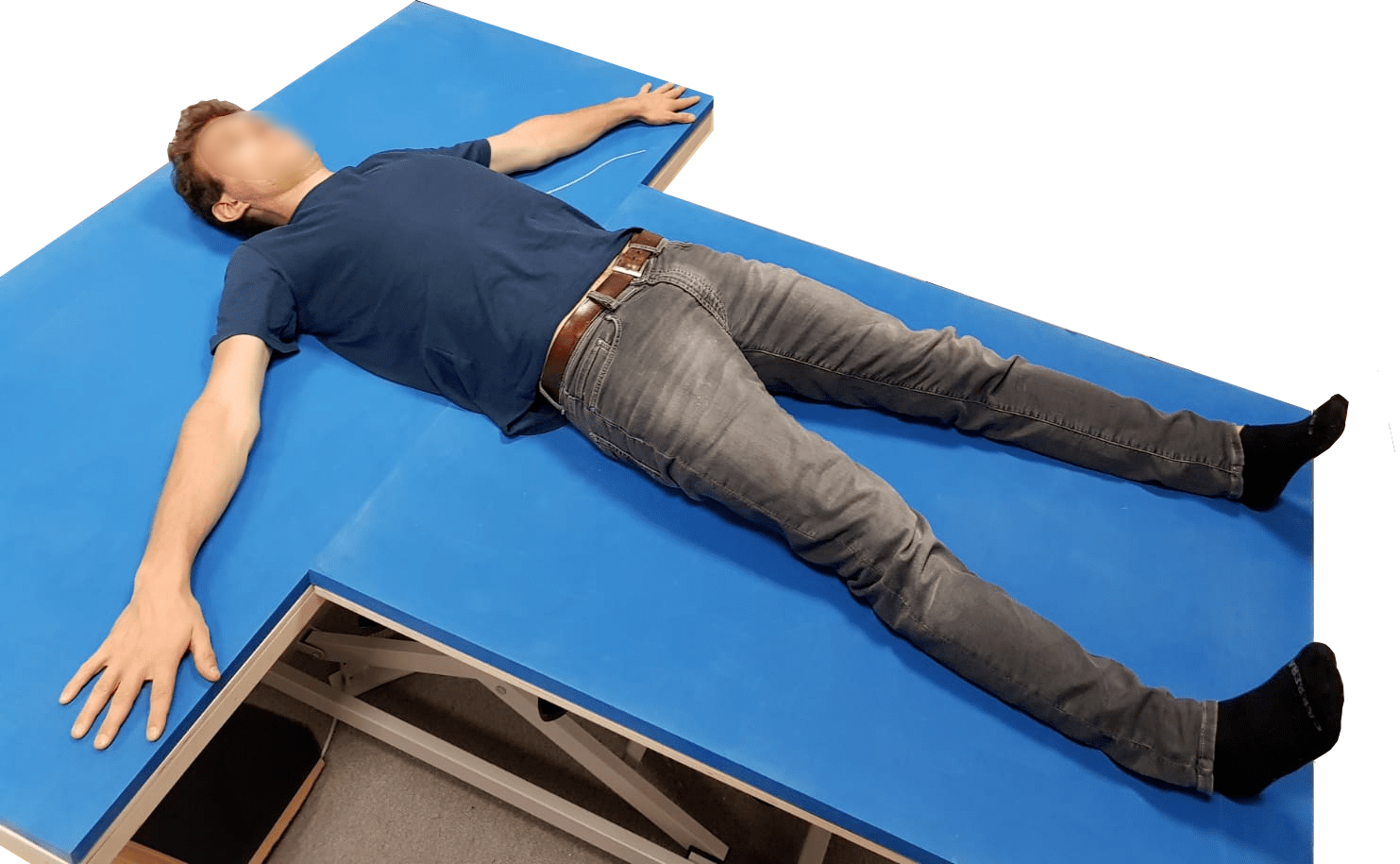#Eurobench is a European academic project that aims to develop a system dedicated to biomedical applications.

The study of human movements and kinematics requires knowledge of the weights and inertia of each part of the body.

Our contribution consists in the development of a measurement system that autonomously estimates these parameters. The system consists of a hospital bed with a low deformation mattress, a ToF camera directed downwards above it and a computer which collects and process the data.

### HOW DOES IT WORK?

ACQUISITION  :

The ToF camera acquires the RGB image and depth image, which are then stored and processed by the local PC.PROCESSING  :

The depth data are processed with a Ransac algorithm, to find the bed surface and isolate the patient’s point cloud from the rest of the environment. The RGB image is processed separately by Open Pose, a Machine Learning algorithm that extrapolates the skeleton and joints from a 2D image. By merging the patient’s point cloud with the 2D coordinates of the joints, it is possible to reconstruct the 3D skeleton and isolate the anatomical parts of the body.VOLUME AND INERTIA COMPUTATION    :

A variation of the traditional Montecarlo approach is used to compute the Volume and the Inertia of each element. The process considers a prismatic element of known volume around the object and generates a large number of points inside it. All the generated points fall within the known volume, but only a part of them also falls within the cloudpoint of the body part taken into consideration. The known volume multiplied by the rate between the points that fell within and out the pointcloud gives the desired volume.

The total body mass is obtained by multiplying the volume by an average density reported in the literature.
Similarly, inertia is calculated. Each time a new point falls within the point cloud, its Euclidean distance from the axes of inertia is calculated. The distance multiplied by the mass of the point provide its inertial contribute. It is important to clarify that the mass of each individual point is given by dividing the mass of the body part by the number of points that have fallen in it.
The overall inertia is calculated as the sum of all the small inertial contributions of these points.